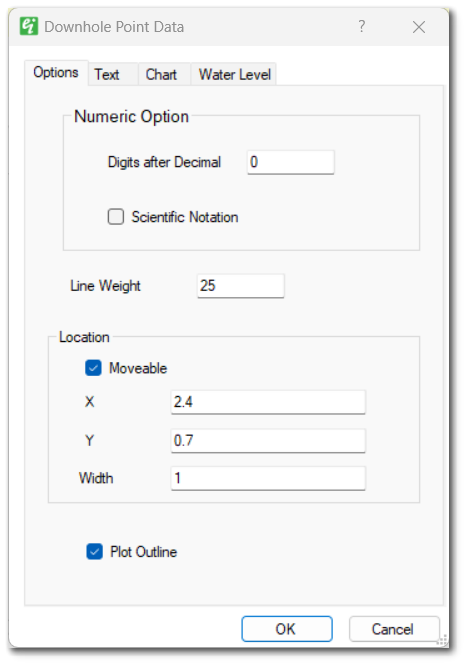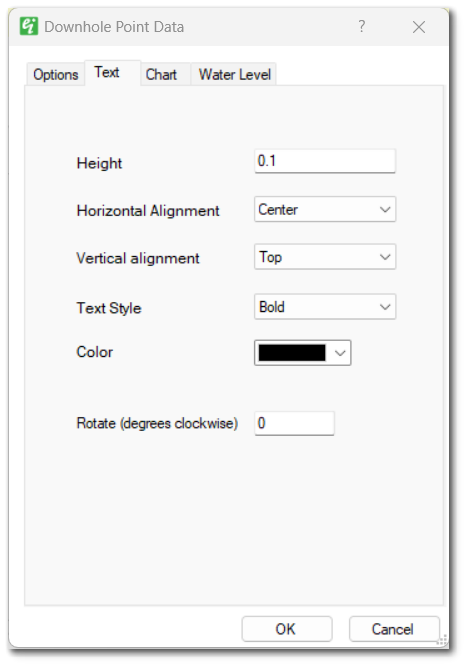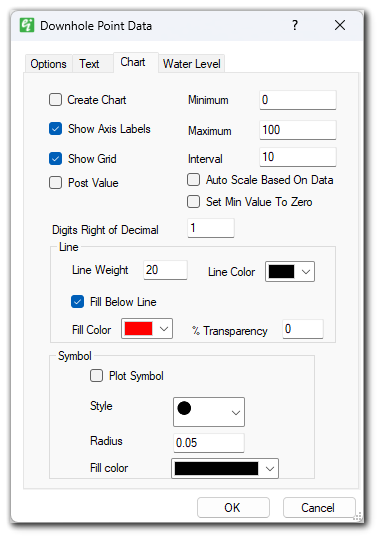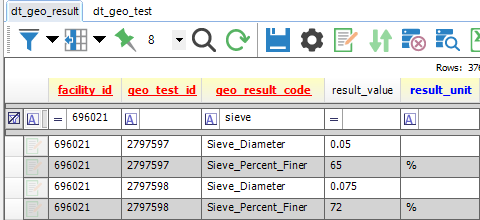﻿ Downhole Point Data

# Downhole Point Data

Navigation:  EnviroInsite > Boring Logs >

# Downhole Point Data

Plots of Downhole Point Data are intended to post or chart numeric values or simply post text descriptions at specified depths.

For EQuIS users, data are queried from DT_DOWNHOLE_POINT_DATA.PARAM_VALUE. DT_COORDINATE.X_COORD and Y_COORD and DT_LOCATION.LOC_TYPE are required to display DT_DOWNHOLE_POINT_DATA in a boring log. In the Boring Log Template Design window, the user is presented with the values of DT_DOWNHOLE_POINT_DATA.PARAM to select the data to include in this plot. If downhole chart is created rather than simply a post of the data, the values in the PARAM_VALUE field will be converted to numeric values. In that case, be sure to not have entered any non-numeric data for records containing the PARAM_VALUE to be plotted. If END_DEPTH is specified, then EnviroInsite will draw a box bounded by START_DEPTH and END_DEPTH.

For non-EQuIS users, the data for these plots are derived from the Point Values table. Each record in the Point Values table has a field for numeric values (Value) and text strings (Text Value). If both numeric values and text values are given for a particular record, then EnviroInsite will always use the text string from the Text Value field. To plot the numeric value, leave the Text Value field empty.

Double-click on the desired downhole point data field to add it to the report. Once in the report, double-click the downhole point data object to open the Downhole Point Data dialog box. Modify the tabs as desired.

Click the OK button to save changes.

Options TabText TabChart TabWater Level Tab

Show Water Level: Option to elect to display the water level on the axis plot as inverted triangle. The depth water must be stored as a field in the Wells table. The field containing the water level is specified in the Log Plotter options dialog box.

Show NAPL Level: Option to elect to display the NAPL level on the axis plot as inverted triangle. The depth to NAPL must be stored as a field in the Wells table (for an EnviroInsite database) or a selection from DT_WATER_TABLE.TYPE (for an EQuIS database). The field containing the depth to NAPL is specified in the Log Plotter Options dialog box.

Data from DT_GEO_RESULT and DT_GEO_TEST are mapped to DT_SAMPLE by DT_GEO_TEST.OBJECT_EUID=DT_SAMPLE.SAMPLE_ID. The data are plotted at depths pulled from DT_GEO_TEST.START_DEPTH if populated, else from DT_SAMPLE.START_DEPTH.

Plasticity Index

These are calculated fields available to be added as Downhole Point Data to EnviroInsite Boring Logs.

The equation used for the Plasticity Index = Liquid Limit - Plastic Limit. These values are in DT_GEO_RESULT.RESULT_VALUE for the records with these corresponding GEO_RESULT_CODEs:

Liquid Limit = Att_LL

Plastic Limit = Att_PL

If the Plastic Limit ≥ Liquid Limit, then the Plasticity Index is displayed as NP (Nonplastic) and plasticity index is not calculated.

Percent Fines

Percent Fines specifications are based on American Society for Testing and Materials (ASTM) standards D4288 and D422 (Material passing the No. 200 sieve [0.075 mm]). For matching GEO_TEST_IDs, the Percent Fines column will plot what is in DT_GEO_RESULT.RESULT_VALUE for record GEO_RESULT_CODE = Sieve_Percent_Finer when the GEO_TEST_ID has a record where DT_GEO_RESULT.RESULT_VALUE = 0.075 mm for GEO_RESULT_CODE = Sieve_Diameter.

Example

In the screenshot below, only 72 would be plotted.Test Type

DT_GEO_TEST.TEST_TYPE is available in the Downhole / Point Data section of the Boring Log Template Design window. Only the test types of Unconf_Compression (UC) or UnconsolidateUndrain (UU) will be plotted as those abbreviations listed.